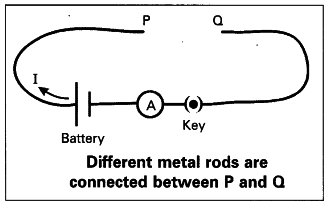# How do you verify that resistance of a conductor

How do you verify that resistance of a conductor is proportional to the length of the conductor for constant cross-section area and temperature ?

Aim : To verify that resistance of a conductor is proportional to the length of the
conductor for constant cross-section area and temperature.
Procedure :

1. Collect iron spokes of different lengths ; with same cross sectional areas.
2. Make a circuit leaving gas between P and Q with a battery, ammeter and plug key.
3. Connect one of the iron spoke say 10 cm length between P and Q.
4. Measure the value of current using ammeter and note the value.
5. Repeat this for other lengths of the iron spokes and note the corresponding values of currents.6. You will observe that the current decreases with increase in the length of the spoke.
7. Thus the resistance of each spoke increases with increase in the length for a constant potential difference.
8. From this, we conclude that the resistance ® of a conductor is directly proportional to its length (l) for constant cross-sectional area.
Therefore, R ∝ L
9. We notice that the resistance was more when the cross - section area was less.
Therefore , R ∝ L/A
Thus we verify l and A